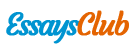# Experiment: Kinematics of Human Motion

Autor:   •  September 29, 2017  •  1,487 Words (6 Pages)  •  224 Views

Page 1 of 6

...

Graph 4: Velocity versus Time Through Time of a Person Moving Toward the Starting Point (at Constant Velocity) [pic 9]

Graph 5: Displacement through Time of a Person Moving Away from the Origin (at Increasing Velocity) [pic 10]

Time (s)

Total Displacement (m)

Average Velocity (m/s)

Instantaneous Velocity (m/s2)

1

0.200

0.200

0.40

2

0.465

0.265

0.53

3

0.840

0.375

0.75

4

1.210

0.370

0.74

5

1.450

0.240

0.48

6

1.755

0.305

0.61

7

2.045

0.290

0.58

8

2.450

0.405

0.81

9

2.880

0.430

0.86

10

3.405

0.525

1.05

Graph 6: Velocity versus Time through Time of a Person Moving Away from the Origin (at Increasing Velocity) [pic 11]

Graph 1 shows the direct relationship of the displacement of a person moving aways from the origin and the elapsed time. On the other hand, if a person moves toward the starting point, the displacement decreases as time elapses.

Graphs 2 and 4, being independent of the displacement, depicted no change of velocity through time.

Graph 5 showing the motion of a person moving away from the origin, neglecting velocity as a variable, depicts a constant increase in displacement through time.

The parabolic line in Graph 6 shows that as the velocity increases through time, the slope or the steepness of the line also increases.

B. Activity 2: Graph Matching

[pic 12]

Graph 6: Relationship of Displacement and Time

[pic 13]

Graph 7: Relationship of Velocity and Time

Graphs 6 and 7 shows how specific the condition is for each point in a graph. The difference of our attempts to the actual lines of the two prepared graphs in the Logger Pro show that the conditions given were not accurately followed. This variation

may be caused by operator errors, instrumental as well as human limitations, and may be resolved by efficiently executing the instructions given and by using a more sensitive Vernier Logger Pro.

C. Activity 3: Graphical Analysis of Motion

Table 1: The Distance Travelled, Average Velocity and Instantaneous Velocity of Aniceto per second as she travelled along a straight line

[pic 14]

Graph 9: Displacement vs. Time (Aniceto)

[pic 15]

Graph 10: Average Velocity of Aniceto[pic 16]

Graph 11: Instantaneous Velocity of Aniceto[pic 17]

D. Activity 4: Reaction Time

Table 2: Reaction Time of Each of the Members of the Group with and without Distractions

Student

Reaction Time (s)

Reaction Time (s) while Calling

0.20

0.24

Alcantara

0.20

0.26

Allam

0.19

0.36

Aniceto

0.19

0.26

The reaction time of each of the members while using cellphone is slower than his reaction time of not. This was computed by the formula: where h is the distance the meter stick has fallen measured from the 50cm mark to where the meter stick was caught and g is the acceleration due to gravity constant, 9.8m/. [pic 18][pic 19]

V. Conclusion

Based from the experiment, we can say that a kinematics describes only the position and change in position of objects but does not deal with the sources of motion. On the other hand, displacement is a vector which points from the intia position of an object to its final position. Meanwhile velocity shows the direction and rate of motion which standard units are meters per second. The average velocity of an object is the total displacement during a period of time divided by the period of time. Acceleration is a vector which shows the direction and magnitude of changes in velocity and bears the standard units of meters per second squared.

...